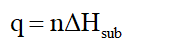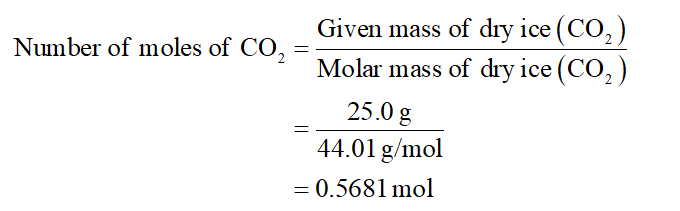# Calculate the amount of heat required to completely sublime 25.0 grams of solid dry ice (CO2) at its sublimation temperature. The heat of sublimation for CO2 is 32.3 kJ/mol.

Question
78 views

Calculate the amount of heat required to completely sublime 25.0 grams of solid dry ice (CO2) at its sublimation temperature. The heat of sublimation for CO2 is 32.3 kJ/mol.

check_circle

Step 1

Given information:

Mass of dry ice = 25.0 g

Heat of sublimation of dry ice ΔHsub = 32.3 kJ/mol

Step 2

The amount of heat (q) required can be expressed in terms of number of moles (n) and heat of sublimation (ΔHsub) for a given sublimation process. The mathematical expression for this is represented as follows:Step 3

First number of moles of dry ice will be calculated as follows:...

### Want to see the full answer?

See Solution

#### Want to see this answer and more?

Solutions are written by subject experts who are available 24/7. Questions are typically answered within 1 hour.*

See Solution
*Response times may vary by subject and question.
Tagged in

### Chemistry